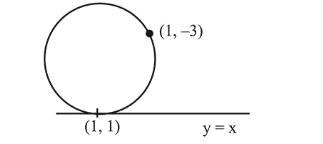# The line x=y touches a circle at the pointQuestion:

The line $x=y$ touches a circle at the point $(1,1)$. If the circle also passes through the point $(1,-3)$, then its radius is:

1. (1) 3

2. (2) $2 \sqrt{2}$

3. (3) 2

4. (4) $3 \sqrt{2}$

Correct Option: , 2

Solution:

Equation of circle which touches the line $y=x$ at $(1,1)$

is, $(x-1)^{2}+(y-1)^{2}+\lambda(y-x)=0$

This circle passes through $(1,-3)$$\therefore 0+16+\lambda(-3-1)=0$

$\Rightarrow 16+\lambda(-4)=0 \Rightarrow \lambda=4$

Hence, equation of circle will be,

$(x-1)^{2}+(y-1)^{2}+4 y-4 x=0$

$\Rightarrow x^{2}+y^{2}-6 x+2 y+2=0$

$\therefore$ Radius $=\sqrt{9+1-2}=2 \sqrt{2}$